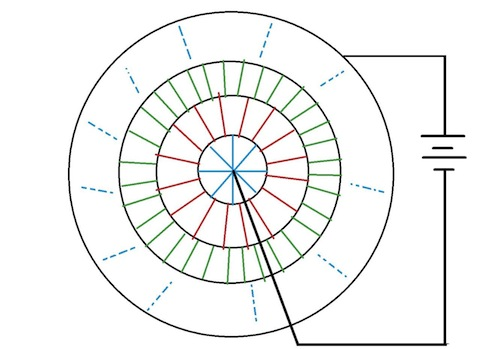In the image, the black lines are connecting wires and colored lines are resistors of $1\, \Omega$ each. The first circle contains 8 resistors, the second one contains 16, the third one contains 32, and so on. There are infinitely many circles of resistors.

A cell is connected between the center and the outermost circle. The equivalent resistance $($in $\Omega)$ between the terminals of the cell is $k.$ What is $100k?$

Assume that the wires (black lines) have negligible resistance.

×